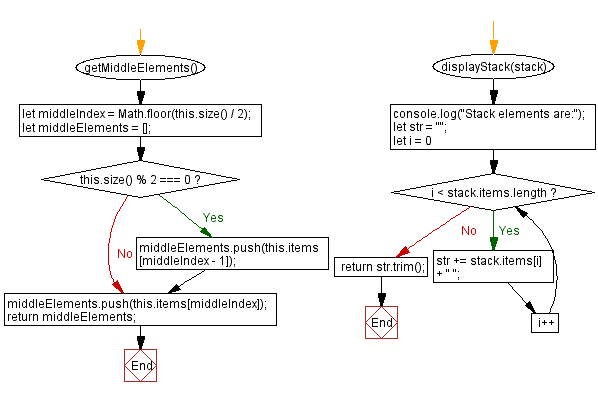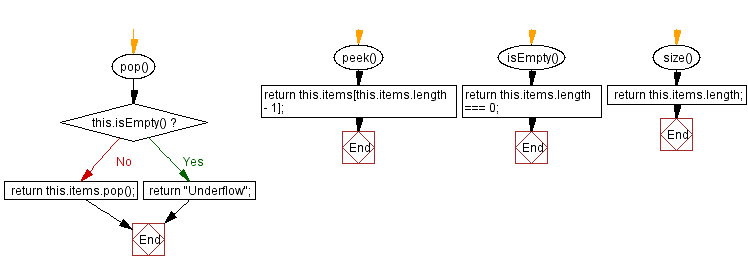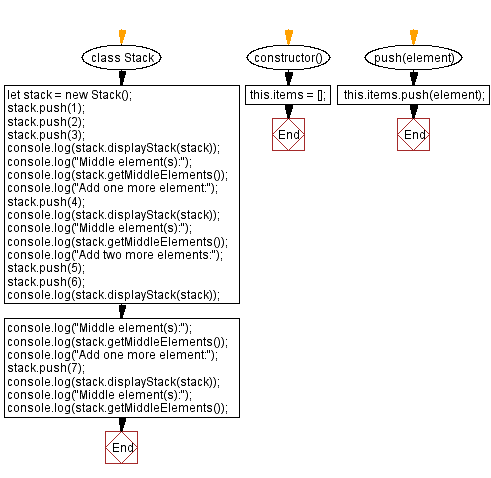# JavaScript Exercises: Middle element(s) of a stack

## JavaScript Stack: Exercise-13 with Solution

Write a JavaScript program to get the middle element(s) of a given stack.

Sample Solution:

JavaScript Code:

``````class Stack {
constructor() {
this.items = [];
}
push(element) {
this.items.push(element);
}
pop() {
if (this.isEmpty()) {
return "Underflow";
}
return this.items.pop();
}
peek() {
return this.items[this.items.length - 1];
}
isEmpty() {
return this.items.length === 0;
}
size() {
return this.items.length;
}
getMiddleElements() {
let middleIndex = Math.floor(this.size() / 2);
let middleElements = [];
if (this.size() % 2 === 0) {
middleElements.push(this.items[middleIndex - 1]);
}
middleElements.push(this.items[middleIndex]);
return middleElements;
}
displayStack(stack) {
console.log("Stack elements are:");
let str = "";
for (let i = 0; i < stack.items.length; i++)
str += stack.items[i] + " ";
return str.trim();
}
}
let stack = new Stack();
stack.push(1);
stack.push(2);
stack.push(3);
console.log(stack.displayStack(stack));
console.log("Middle element(s):");
console.log(stack.getMiddleElements());
stack.push(4);
console.log(stack.displayStack(stack));
console.log("Middle element(s):");
console.log(stack.getMiddleElements());
stack.push(5);
stack.push(6);
console.log(stack.displayStack(stack));
console.log("Middle element(s):");
console.log(stack.getMiddleElements());
stack.push(7);
console.log(stack.displayStack(stack));
console.log("Middle element(s):");
console.log(stack.getMiddleElements());
```
```

Sample Output:

```Stack elements are:
1 2 3
Middle element(s):

Stack elements are:
1 2 3 4
Middle element(s):
[2,3]
Stack elements are:
1 2 3 4 5 6
Middle element(s):
[3,4]
Stack elements are:
1 2 3 4 5 6 7
Middle element(s):

```

Flowchart:Live Demo:

See the Pen javascript-stack-exercise-13 by w3resource (@w3resource) on CodePen.

Improve this sample solution and post your code through Disqus

Stack Previous: Rotate the stack elements to the right.
Stack Exercises Next: Remove a specific element from a stack.

What is the difficulty level of this exercise?

Test your Programming skills with w3resource's quiz.

﻿